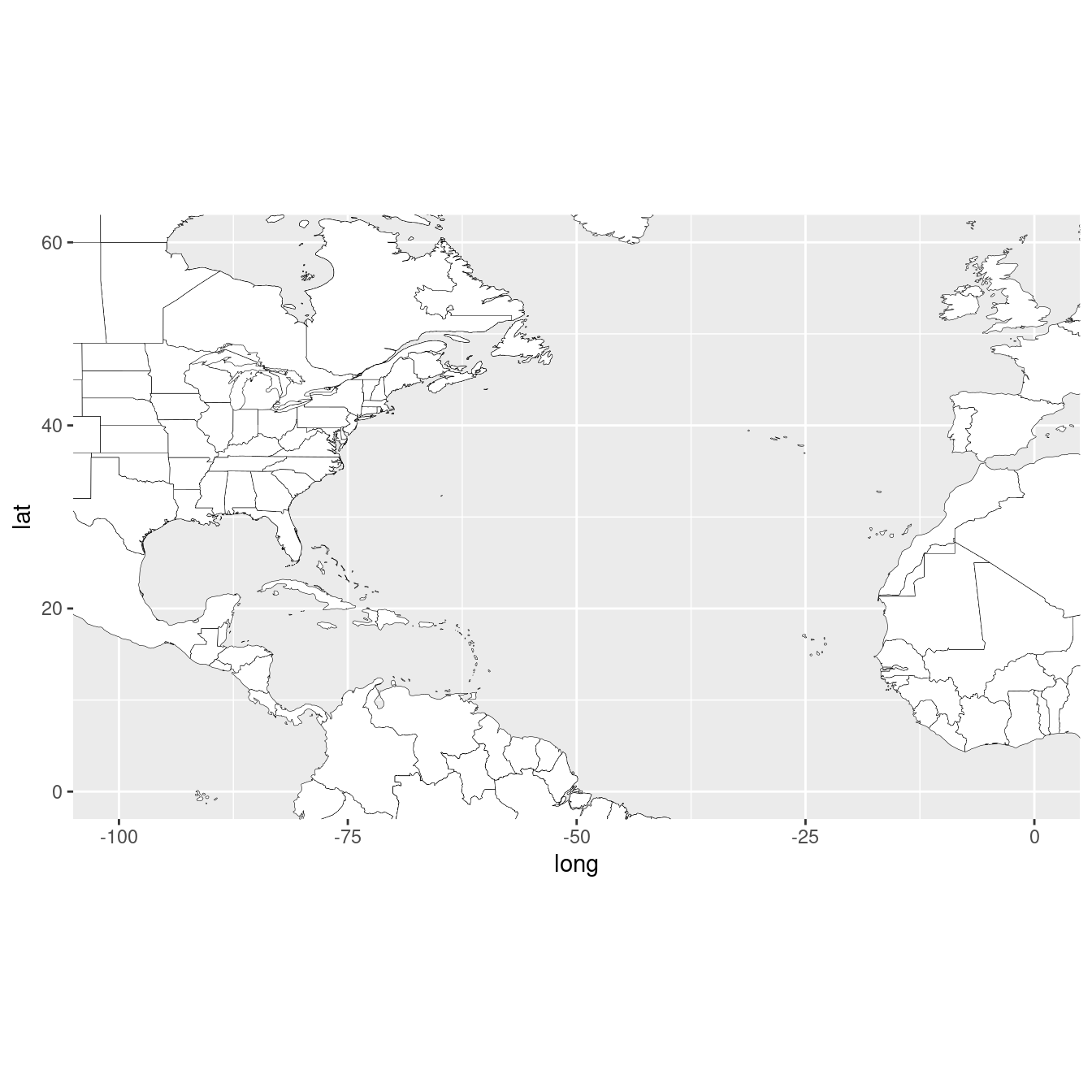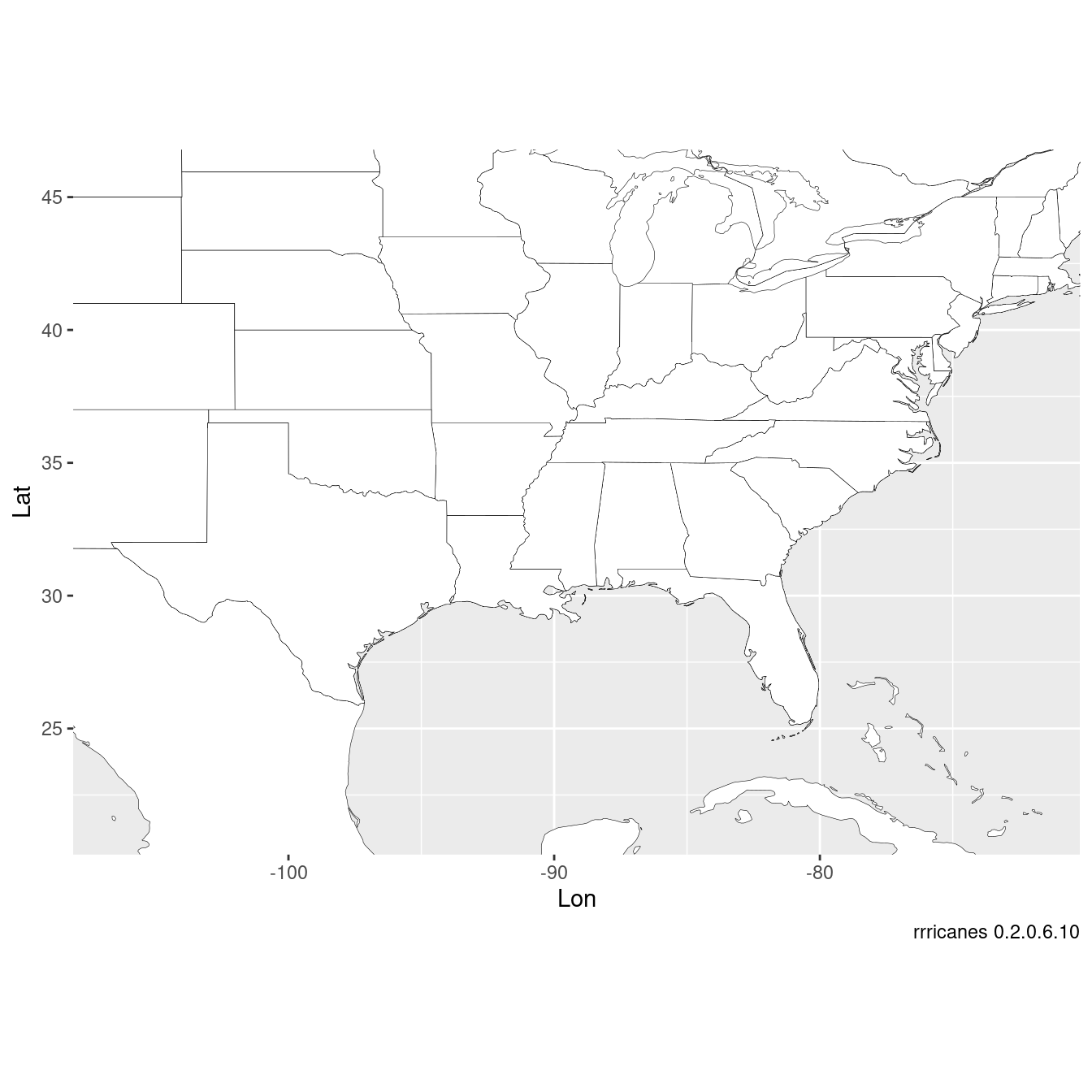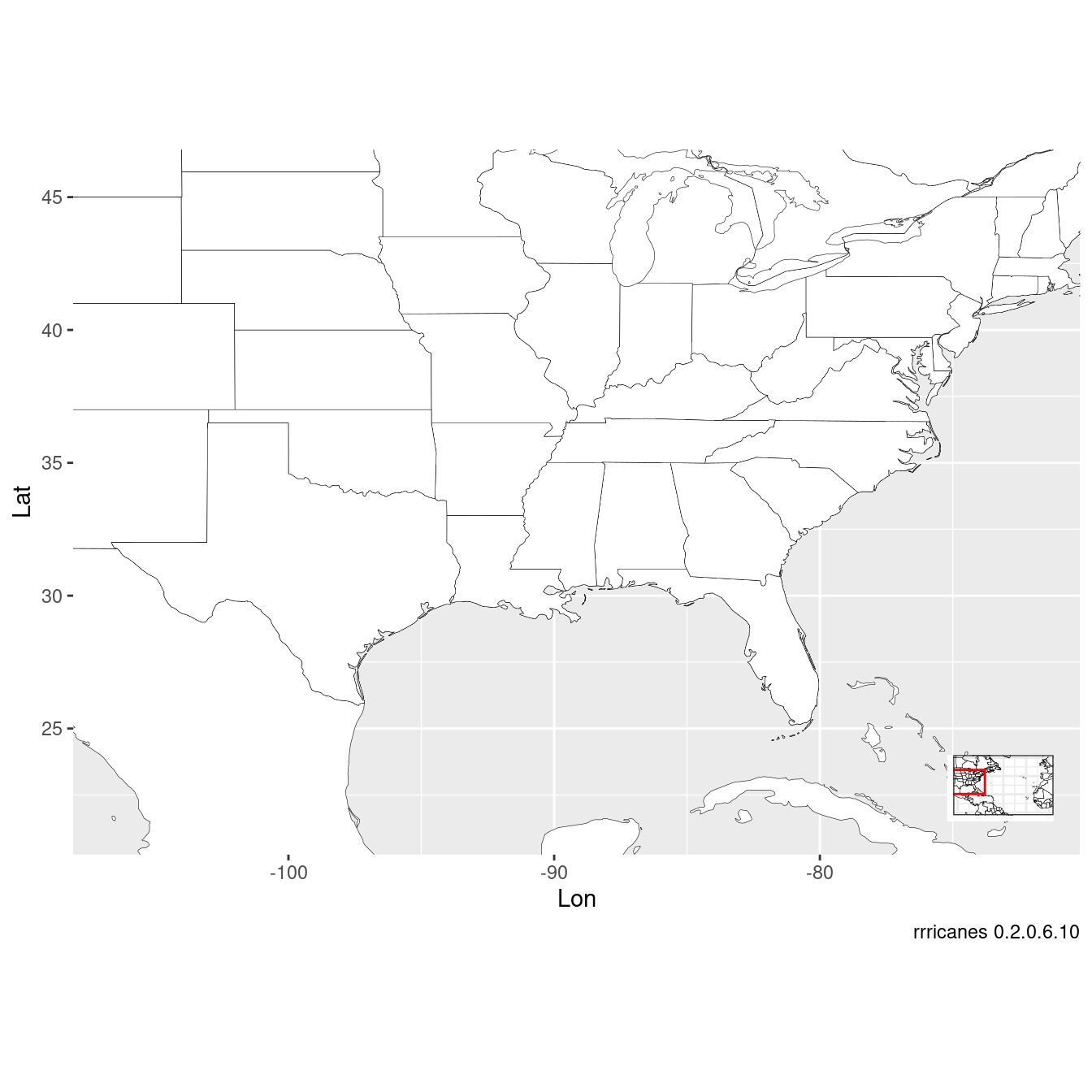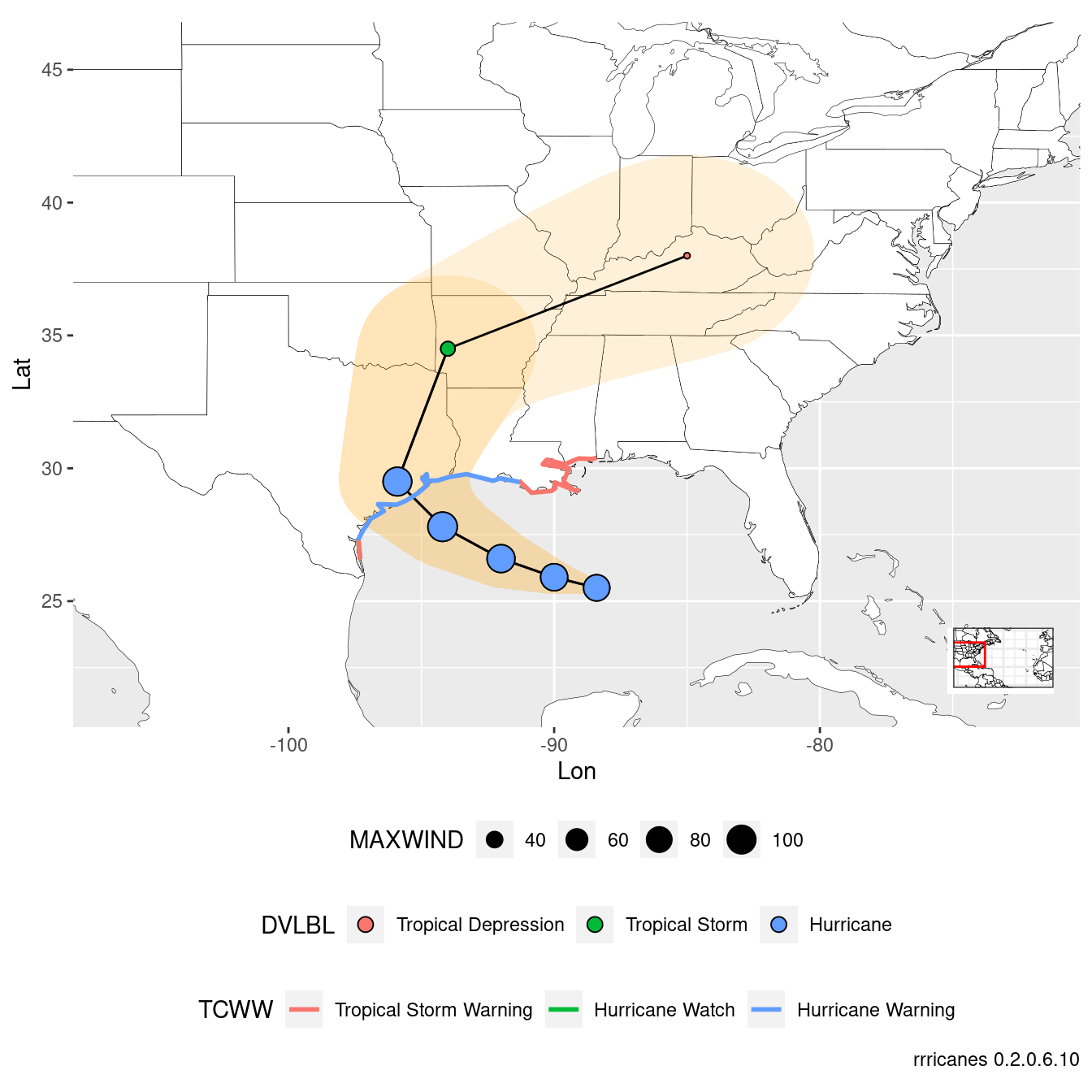``````library(dplyr)
library(ggplot2)
library(rrricanes)
library(rrricanesdata)
library(sp)``````
``````key <- "AL092008"
``fstadv <- fstadv %>% filter(Key == key, Adv <= adv)``

### GIS Advisory Forecast Track, Cone of Uncertainty, and Watches/Warnings

``gis_adv <- gis_advisory(key = key, advisory = adv) %>% gis_download()``
``````## Warning in OGRSpatialRef(dsn, layer, morphFromESRI = morphFromESRI, dumpSRS =
## dumpSRS, : Discarded ellps Sphere in Proj4 definition: +proj=longlat +R=6371200
## +no_defs``````
``````## Warning in OGRSpatialRef(dsn, layer, morphFromESRI = morphFromESRI, dumpSRS
## = dumpSRS, : Discarded datum Not_specified_based_on_Authalic_Sphere in Proj4
## definition: +proj=longlat +R=6371200 +no_defs``````
``````## Warning in showSRID(wkt2, "PROJ"): Discarded ellps Sphere in Proj4 definition:
## +proj=longlat +R=6371200 +no_defs +type=crs``````
``````## Warning in showSRID(wkt2, "PROJ"): Discarded datum Not specified (based on
## Authalic Sphere) in Proj4 definition``````
``````## OGR data source with driver: ESRI Shapefile
## Source: "/tmp/RtmpYDYHD5", layer: "al092008.042_5day_lin"
## with 2 features
## It has 9 fields``````
``````## Warning in OGRSpatialRef(dsn, layer, morphFromESRI = morphFromESRI, dumpSRS =
## dumpSRS, : Discarded ellps Sphere in Proj4 definition: +proj=longlat +R=6371200
## +no_defs``````
``````## Warning in OGRSpatialRef(dsn, layer, morphFromESRI = morphFromESRI, dumpSRS
## = dumpSRS, : Discarded datum Not_specified_based_on_Authalic_Sphere in Proj4
## definition: +proj=longlat +R=6371200 +no_defs``````
``````## Warning in showSRID(wkt2, "PROJ"): Discarded ellps Sphere in Proj4 definition:
## +proj=longlat +R=6371200 +no_defs +type=crs``````
``````## Warning in showSRID(wkt2, "PROJ"): Discarded datum Not specified (based on
## Authalic Sphere) in Proj4 definition``````
``````## OGR data source with driver: ESRI Shapefile
## Source: "/tmp/RtmpYDYHD5", layer: "al092008.042_5day_pgn"
## with 2 features
## It has 9 fields``````
``````## Warning in OGRSpatialRef(dsn, layer, morphFromESRI = morphFromESRI, dumpSRS =
## dumpSRS, : Discarded ellps Sphere in Proj4 definition: +proj=longlat +R=6371200
## +no_defs``````
``````## Warning in OGRSpatialRef(dsn, layer, morphFromESRI = morphFromESRI, dumpSRS
## = dumpSRS, : Discarded datum Not_specified_based_on_Authalic_Sphere in Proj4
## definition: +proj=longlat +R=6371200 +no_defs``````
``````## Warning in showSRID(wkt2, "PROJ"): Discarded ellps Sphere in Proj4 definition:
## +proj=longlat +R=6371200 +no_defs +type=crs``````
``````## Warning in showSRID(wkt2, "PROJ"): Discarded datum Not specified (based on
## Authalic Sphere) in Proj4 definition``````
``````## OGR data source with driver: ESRI Shapefile
## Source: "/tmp/RtmpYDYHD5", layer: "al092008.042_5day_pts"
## with 13 features
## It has 20 fields``````
``````## Warning in OGRSpatialRef(dsn, layer, morphFromESRI = morphFromESRI, dumpSRS =
## dumpSRS, : Discarded ellps Sphere in Proj4 definition: +proj=longlat +R=6371200
## +no_defs``````
``````## Warning in OGRSpatialRef(dsn, layer, morphFromESRI = morphFromESRI, dumpSRS
## = dumpSRS, : Discarded datum Not_specified_based_on_Authalic_Sphere in Proj4
## definition: +proj=longlat +R=6371200 +no_defs``````
``````## Warning in showSRID(wkt2, "PROJ"): Discarded ellps Sphere in Proj4 definition:
## +proj=longlat +R=6371200 +no_defs +type=crs``````
``````## Warning in showSRID(wkt2, "PROJ"): Discarded datum Not specified (based on
## Authalic Sphere) in Proj4 definition``````
``````## OGR data source with driver: ESRI Shapefile
## Source: "/tmp/RtmpYDYHD5", layer: "al092008.042_ww_wwlin"
## with 5 features
## It has 10 fields``````

Get bounding box of the forecast polygon.

``bbox <- bbox(gis_adv\$al092008.042_5day_pgn)``

Generate a base plot of the Atlantic ocean.

``(bp <- al_tracking_chart(color = "black", fill = "white", size = 0.1, res = 50))``
``````## Regions defined for each Polygons
## Regions defined for each Polygons``````
``## Coordinate system already present. Adding new coordinate system, which will replace the existing one.``I like to add a little cushion for the map inset and forecast cone data.

``````lat_min <- bbox[2,1] - 5
lat_max <- bbox[2,2] + 5
lon_min <- bbox[1,1] - 10
lon_max <- bbox[1,2] + 10``````

Build a thin tracking map for the inset.

``````bp_inset <- ggplotGrob(bp +
geom_rect(mapping = aes(xmin = lon_min, xmax = lon_max,
ymin = lat_min, ymax = lat_max),
color = "red", alpha = 0) +
theme_bw() +
theme(axis.title = element_blank(),
axis.ticks = element_blank(),
axis.text.x = element_blank(),
axis.text.y = element_blank(),
plot.margin = margin(0, 0, 0, 0, "pt")))``````

Modify original `bp` zoomed in on our area of interest.

``````(bp <- bp +
coord_equal(xlim = c(lon_min, lon_max),
ylim = c(lat_min, lat_max)) +
scale_x_continuous(expand = c(0, 0)) +
scale_y_continuous(expand = c(0, 0)) +
labs(x = "Lon",
y = "Lat",
caption = sprintf("rrricanes %s", packageVersion("rrricanes"))))``````
``## Coordinate system already present. Adding new coordinate system, which will replace the existing one.``Combine `bp` and `bp_inset` to finalize initial base plot. `bp` will be a base plot without the inset. `bpi` will have the inset.

``````(bpi <- bp + annotation_custom(grob = bp_inset, xmin = lon_max - 5,
xmax = lon_max - 1, ymin = -Inf,
ymax = lat_min + 5))``````Lines and Polygons spatial dataframes can be helpfully converted using `shp_to_df`. The original spatial dataframes can be plotted directly in `ggplot2` but, to my understanding, access to the other variables are not available.

``````# Convert object SpatialLinesDataframe to dataframe
# Convert object SpatialPolygonsDataframe to dataframe

Points dataframes can just be converted with `tibble::as_data_frame`.

``````# Convert object SpatialPointsDataframe to dataframe
``````## Warning: `as_data_frame()` was deprecated in tibble 2.0.0.
## The signature and semantics have changed, see `?as_tibble`.
## This warning is displayed once every 8 hours.
## Call `lifecycle::last_lifecycle_warnings()` to see where this warning was generated.``````

Modify `shp_storm_pts\$DVLBL` with full strings and ordered factor.

``````shp_storm_pts\$DVLBL <- factor(shp_storm_pts\$DVLBL,
levels = c("D", "S", "H"),
labels = c("Tropical Depression",
"Tropical Storm",
"Hurricane"))``````

Same with `shp_storm_pts\$TCWW`:

``````shp_storm_ww\$TCWW <- factor(shp_storm_ww\$TCWW,
levels = c("TWA", "TWR", "HWA", "HWR"),
labels = c("Tropical Storm Watch",
"Tropical Storm Warning",
"Hurricane Watch",
"Hurricane Warning"))``````
``````bpi + geom_polygon(data = shp_storm_pgn,
aes(x = long, y = lat, group = group),
alpha = 0.15, fill = "orange") +
geom_path(data = shp_storm_lin, aes(x = long, y = lat, group = group)) +
geom_point(data = shp_storm_pts, aes(x = LON, y = LAT, fill = DVLBL,
shape = DVLBL, size = MAXWIND)) +
geom_path(data = shp_storm_ww, aes(x = long, y = lat, color = TCWW,
group = group), size = 1) +
scale_shape_manual(values = c(21, 21, 21, 21)) +
guides(shape = guide_legend(override.aes = list(size = 3)),
size = guide_legend(nrow = 1)) +
theme(legend.position = "bottom",
legend.box = "vertical")``````Very often, areas that are under a hurricane watch may also be under a tropical storm warning. The chart above does not show the hurricane watch area.